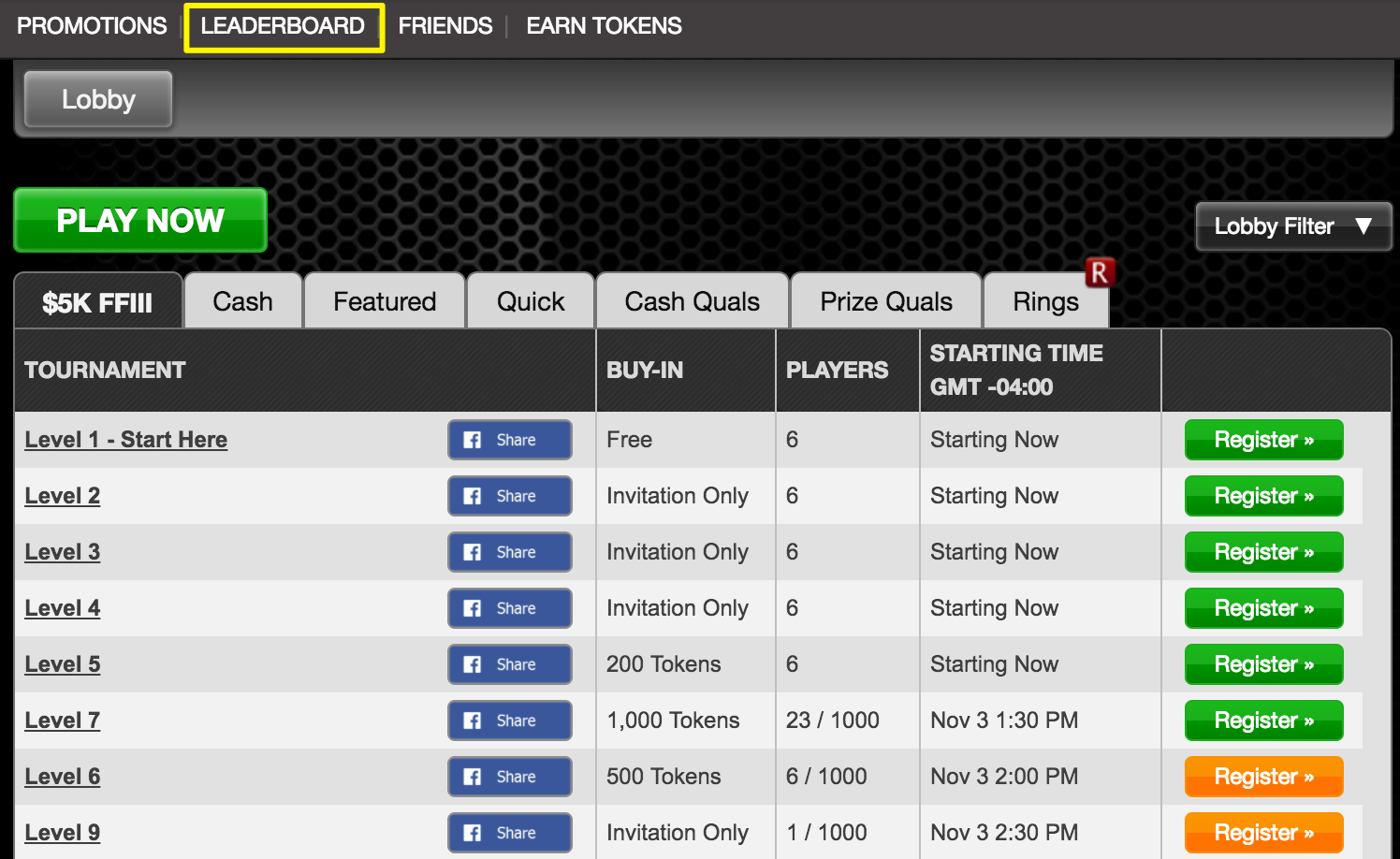If you rank in a tourney you will receive points towards the Tournament Leaderboard standings, based on the number of entrants, the tournament buy-in, and place of finish. If you lose your chips before late registration expires or if you time out for inactivity, you will not have a rank and will not be calculated into the leaderboard standings.

Weekly leaderboards start Sunday at 12am EST and end on Saturday at 11:59pm EST.

Monthly leaderboards start on the 1st of the month at 12am EST and end on the last day of the month at 11:59pm EST.

Prizes won for placing in the leaderboard may vary from week to week and month to month. Please check the leaderboard for the current cycles' prizes. You can access the leaderboard at any point by clicking the "LEADERBOARDS" link in the navigation bar at the top of the app lobby:The formula used to determine the total leaderboard points (LBP) awarded to a player for any given tournament is:

Points = 10 * (sqrt(n)/sqrt(f)) * [1+|log(b+0.25)|*p]

Where:

n is the number of entrants
f is the place of finish (f=1 for the first-place finisher, and so on)

p is the prize type (1 = Invitation; 2 = Tokens; 5 = Cash/External)

Example 1: A player takes first place in a \$1000 tournament with a field of 200 players and a 2000 Token buy-in. Here:

n = 200, f=1, b=2000, p=5

The total points awarded to this player are:

= 10 * [sqrt(n)/sqrt(f)] * [1+|log(b+0.25)| * p]

= 10 * [sqrt(200)/sqrt(1)] * [1+|log(2000+0.25)| * 5]
= 2476 LBP

Example 2: A player takes first place in a freeroll cash tourney with a field of 1200 players. Here:

n = 1200, f=1, b=0, p=5

The total points awarded to this player are:

= 10 * [sqrt(n)/sqrt(f)] * [1+|log(b+0.25)| * 5]
= 10 * [sqrt(1200)/sqrt(1)] * [1+|log(0+0.25)| * 5]
= 696 LBP

Example 3: A player takes first place in a Token tournament with a field of 10 players and a 55 Token buy-in. Here:

n = 10, f=1, b = 55, p = 2

The total points awarded to this player are:

= 10 * [sqrt(n)/sqrt(f)] * [1+|log(b+0.25)| * p]
= 10 * [sqrt(10)/sqrt(1)] * [1+|log(55+0.25)| * 2]
= 142 LBP

Example 4: A player takes first place in a Level SNG tournament with a free buy-in, a field of 6 players and a prize of Tokens + Invitation. Here:

n = 6, f=1, b = 0, p = 3

The total points awarded to this player are:

= 10 * [sqrt(n)/sqrt(f)] * [1+|log(b+0.25)| * p]
= 10 * [sqrt(6)/sqrt(1)] * [1+|log(0+0.25)| * 3]
= 20

Example 5: A player takes first place in a Level SNG tournament with a 200 Token buy-in, a field of 6 players and a prize of Tokens + Invitation. Here:

n = 6, f=1, b = 200, p = 3

The total points awarded to this player are:

= 10 * [sqrt(n)/sqrt(f)] * [1+|log(b+0.25)| * p]
= 10 * [sqrt(6)/sqrt(1)] * [1+|log(200+0.25)| * 3]
= 194 LBP

Example 6: A player takes first place in a SNG tournament with a 40 Token buy-in, a field of 10 players and a cash prize. Here:

The total points awarded to this player are:

= 10 * [sqrt(n)/sqrt(f)] * [1+|log(b+0.25)| * p]
= 10 * [sqrt(10)/sqrt(1)] * [1+log|40+0.25)| * 5]
= 285 LBP